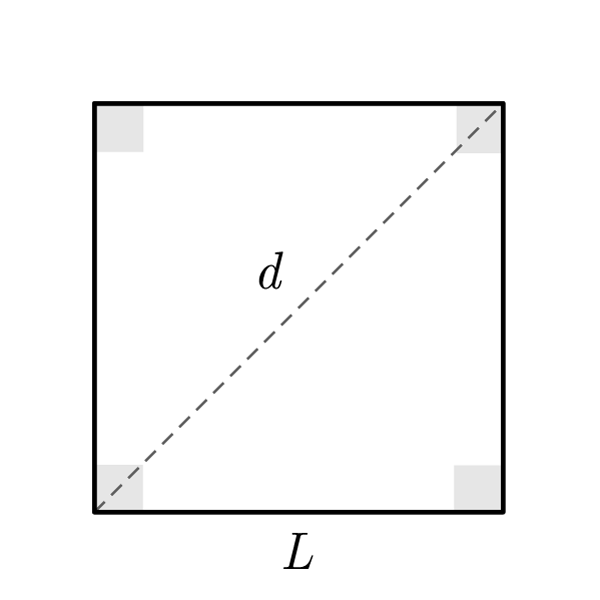# Square

 Side $$S$$ Diagonal $$d$$
$$2p = S \times 4$$
Perimeter
$$S = \frac{2p}{4}$$
$$A = {S}^{2}$$
Area
$$S = \sqrt{A}$$
$$d = S \sqrt{2}$$
Diagonal
$$S = \frac{d}{\sqrt{2}}$$
$$A = \frac{{d}^{2}}{2}$$
Area
$$d = \sqrt{2A}$$

## Definition

A square is a polygon with four sides and four congruent angles (right angles).

## Properties

1. Four congruent sides
2. Four congruent right angles
3. Diagonals are perpendicular
4. The diagonal makes two right congruent triangles. In particular every triangle has angles of 45°, 45°, 90°## Square Formulas

Data Formula
Perimeter 2p = S × 4
Area A = S2
Side S = 2p / 4
Side S = √A
Diagonal d = S × √2
Side S = d / √2
Area A = d2 / 2
Diagonal d = √(2A)